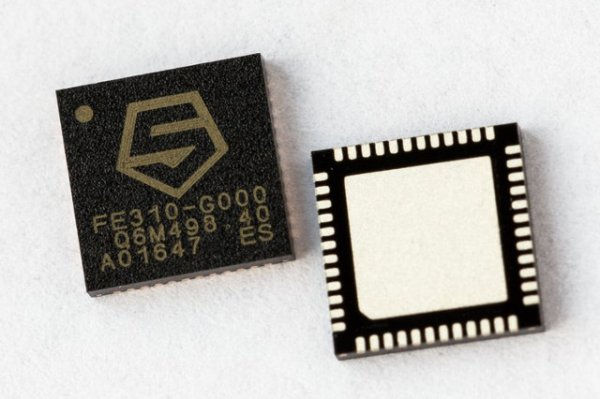# Arduino MKR1000/Zero Neopixel Throwie

A WiFi controlled NeoPixel display. Designed for the MKR1000 but should work for the Zero too. A little rough around the edges but works!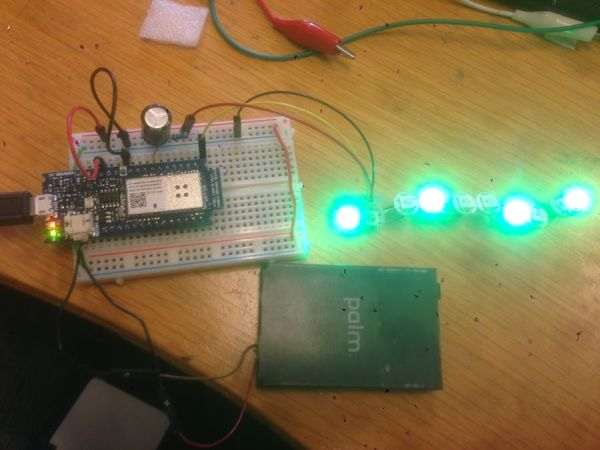## Things used in this project

### Hardware components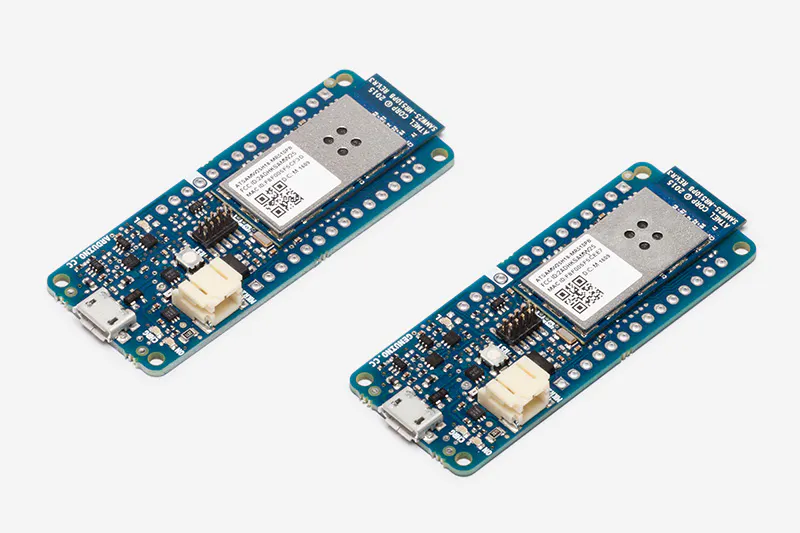Arduino MKR1000
×1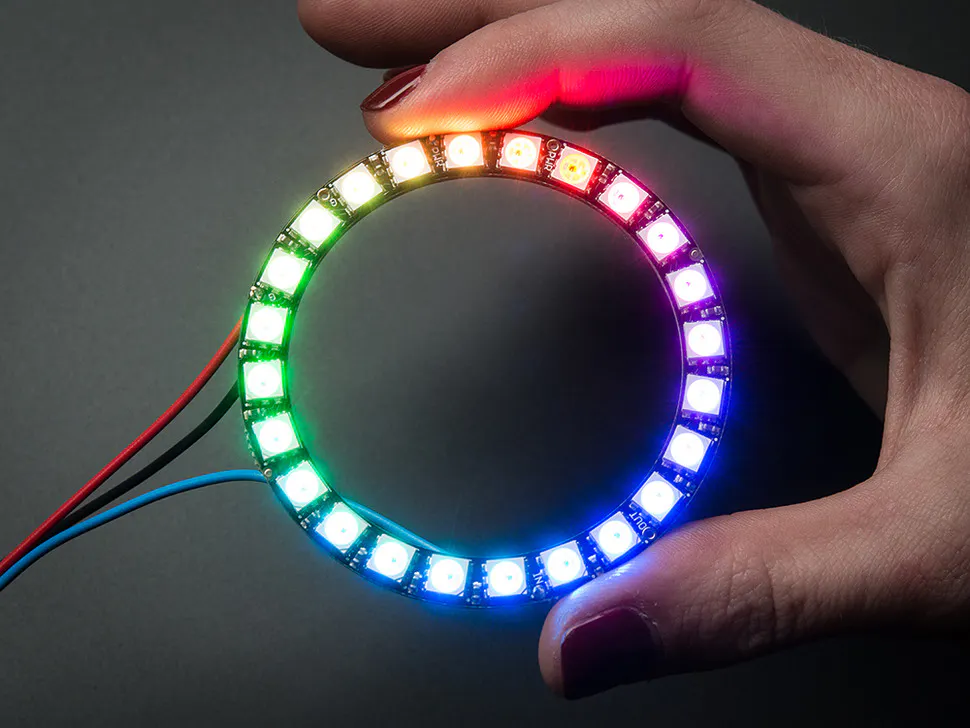Adafruit NeoPixel Ring: WS2812 5050 RGB LED
×1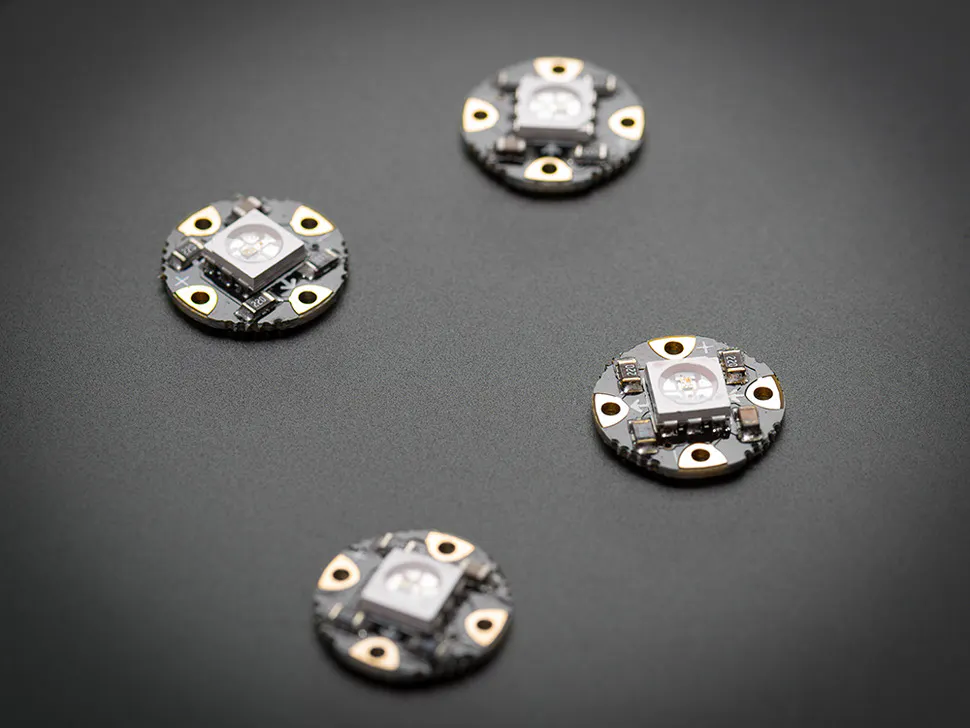Adafruit Flora RGB Neopixel LEDs- Pack of 4
×1×1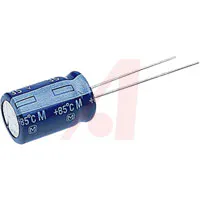Capacitor 1000 µF
×1Jumper wires (generic)
×1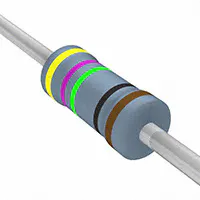Resistor 475 ohm 470 Ohm resistor will do
×1

## Story### MKR1000 Circuit### 1. Picture of Circuit

A photograph of the circuit assembled### 2 . Crude Drawing of Schematic

As MKR1000 not available on Fritzing and would take too long to make on Eagle I drew this up very quickly.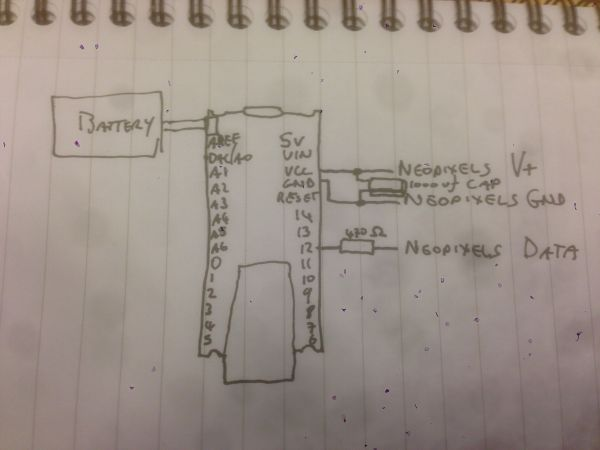## Code

### WiFi101_AP_Neopixels.ino

Arduino

Copy and Paste
```/*
WiFi101_AP_Neopixels - built for the MKR1000 but should work for Zero too
Created by Colin Conway (Collie147 on Arduino.cc) 25/02/2016
Works connected to Wifi hotspot/router and on it's own as a 'Throwie'
*
*
created 25 Nov 2012
by Tom Igoe
And various bits and pieces from the ESP8266.com boards
*/

#include <WiFi101.h>
#define PIN            12 //sets the pin on which the neopixels are connected
#define NUMPIXELS      10 //defines the number of pixels in the strip
#define interval       50 //defines the delay interval between running the functions
#define wifiRetryTimes 0 //number of times to try the wifi router before going into AP mode (set to 0 if AP only)

uint32_t red = pixels.Color(255, , );
uint32_t blue = pixels.Color(, , 255);
uint32_t green = pixels.Color(, 255, );
uint32_t pixelColour;
uint32_t lastColor;
float activeColor[] = {255, , };//sets the default color to red

IPAddress apIP(192, 168, , 150);//not yet working in AP mode - if using a router and you would like a static IP address change this to the address (make sure on the same subnet)!
IPAddress netMsk(255, 255, 255, );//not yet working in AP mode
IPAddress gw(192, 168, , 1);//not yet working in AP mode
IPAddress dns(192, 168, , 1);//not yet working in AP mode
String IP;
char APssid[] = "MKR1000";  //SSID when in AP mode
char APpass[] = "MKR1000";  //Password when in AP MODE (NOT CURRENTLY WORKING)
int keyIndex = ;
int status = WL_IDLE_STATUS;
WiFiServer server(80);

/*HTML code is split into 4 strings as it doesnt seem to be able to handle strings over 1500 or so chars*/
const char html1[] PROGMEM =
"<!DOCTYPE html><html><head><meta http-equiv='X-UA-Compatible' content='IE=Edge'><meta charset='utf-8'><title>Neopixel Color Picker</title>"
".red{background:red;color:white;}.green{background:#0C0;color:white;}.blue{background:blue;color:white;}"
".white{background:white;color:black;border:1px solid black;}.off{background:#666;color:white;}.colorPicker{background:white;color:black;}.colorWipe{font-size:40px;  background:linear-gradient(to right, red, #0C0, blue);}"
".theatreChase{font-size:40px;background:linear-gradient(to right, red, black, red, black, #0C0, black, #0C0, black, blue, black, blue);}"
".rainbow{font-size:40px;background:red;background:linear-gradient(to right, red, orange, yellow, green, blue, indigo, violet,red, orange, yellow, green, blue, indigo, violet);}"
".rainbowCycle{font-size:40px;background:red;background:linear-gradient(to right, red, orange, yellow, green, blue, indigo, violet);}"
".rainbowChase{font-size:40px;background:red;background:linear-gradient(to right, red, black, orange, black, yellow, black, green, black, blue, black, indigo, black, violet);}";

const char html2[] PROGMEM =
".breathe{background:blue;color:white;}.cylon{background:red;color:black;}.heartbeat{background:red;color:white;}.XMAS{font-size:40px;background:red;background:linear-gradient(to right, red, green, red, green, red, green, red, green, red, green, red, green);}"
"<script type='text/javascript'>";

const char html3[] PROGMEM =
"function ResetWebpage(){ if (window.location.href != 'http://#IPADDRESS/'){    window.open ('http://#IPADDRESS/','_self',true) }};" //change the website value here to your static website
"function myFunction(){ document.getElementById('brightnessLevel').submit();}</script>"
"<body onload='ResetWebpage()'><table><tr><td width='100'><div class='TGT00'></div></td><td><a class='bt red' href='/L00?v=1'>Red</a></td><td><a class='bt colorWipe' href='/L01?v=1'>Color Wipe</a></td><td><div class='TGT01'></div></td></tr>"
"<tr><td><div class='TGT02'></div></td><td><a class='bt green' href='/L02?v=1'>Green</a></td><td><a class='bt theatreChase' href='/L03?v=1'>Theatre Chase</a></td><td><div class='TGT03'></div></td></tr>"
"<tr><td><div class='TGT04'></div></td><td><a class='bt blue' href='/L04?v=1'>Blue</a></td><td><a class='bt rainbow' href='/L05?v=1'>Rainbow</a></td><td><div class='TGT05'></div></td></tr>"
"<tr><td><div class='TGT06'></div></td><td><a class='bt white' href='/L06?v=1'>White</a></td><td><a class='bt rainbowChase' href='/L07?v=1'>Rainbow Chase</a></td><td><div class='TGT07'></div></td></tr>";

const char html4[] PROGMEM =
"<tr><td><div class='TGT08'></div></td><td><a class='bt cylon' href='/L08?v=1'>Cylon Chaser</a></td><td><a class='bt rainbowCycle' href='/L09?v=1'>Rainbow Cycle</a></td><td><div class='TGT09'></div></td></tr>"
"<tr><td><div class='TGT10'></div></td><td><a class='bt breathe' href='/L10?v=1'>Breathe</a></td><td><a class='bt heartbeat' href='/L11?v=1'>Heartbeat</a></td><td><div class='TGT11'></div></td></tr>"
"<tr><td><div class='TGT12'></div></td><td><a class='bt XMAS' href='/L12?v=1'>Christmas</a></td><td><a class='bt ALL' href='/L13?v=1'>Cycle ALL</a></td><td><div class='TGT13'></div></td></tr>"
"<tr><td><div class='TGT14'></div></td><td><a class='bt off' href='/L14?v=1'>Off</a></td><td><form id='brightnessLevel'><input type='range' name='bright' max='255' min='0' value='#BRIGHT' onchange='myFunction()' class='bt off'></form></td></tr>"
"</table></body></html>";

String sendHtml3 = html3;//create a string version of the html3 to sub values into
String sendHtml4 = html4;//create a string version of the html4 to sub values into
String currentLine; //string returned by the client connected
boolean NeoState[] = {false, false, false, false, false, false, false, false, false, false, false, false, false, false, true}; //Active Neopixel Function (off by default)

int neopixMode = ; //sets a mode to run each of the functions
long previousMillis = ; // a long value to store the millis()
long lastAllCycle = ; // last cycle in the ALL() function
long previousColorMillis = ; // timer for the last color change
int i = ; //sets the pixel number in newTheatreChase() and newColorWipe()
int CWColor = ; //sets the newColorWipe() color value 0=Red, 1=Green, 2=Blue
int j; //sets the pixel to skip in newTheatreChase() and newTheatreChaseRainbow()
int cycle = ;//sets the cycle number in newTheatreChase()
int TCColor = ;//sets the color in newTheatreChase()
int l = ; //sets the color value to send to Wheel in newTheatreChaseRainbow() and newRainbow()
int m = ; //sets the color value in newRainbowCycle()
int n = 2; //sets the pixel number in cyclonChaser()
int breather = ; //sets the brightness value in breather()
boolean dir = true; //sets the direction in breather()-breathing in or out, and cylonChaser()-left or right
boolean beat = true; //sets the beat cycle in heartbeat()
int beats = ; //sets the beat number in heartbeat()
int brightness = 150; //sets the default brightness value
int o = ; //christmas LED value
int q = 5; // values for the All() function
uint32_t lastAllColor = ; // last color displayed in the All() function

void setup() {
pixels.begin(); //starts the neopixels
pixels.setBrightness(brightness); // sets the inital brightness of the neopixels
writeLEDS(, , ); //sets all the pixels to off
Serial.begin(9600);
// Connect to WPA/WPA2 network. Change this line if using open or WEP network:
Serial.print(F("Creating Network named: "));
Serial.println(ssid);                   // print the network name (SSID);
if (WiFi.status() == WL_NO_SHIELD) {
Serial.println(F("WiFi shield not present"));
while(true);  // don't continue
}
WiFi.config(apIP);  //Sets IP address to static.  Comment out if not needed (not yet working in AP mode)
//WiFi.config(apIP, dns, gw, netMsk); //not yet working in AP mode
while ( status != WL_CONNECTED) {
for (int x = ; x <= wifiRetryTimes; x++)
{
Serial.print(F("Attempting to connect to Network named: "));
Serial.println(ssid);                   // print the network name (SSID);

// Connect to WPA/WPA2 network. Change this line if using open or WEP network:
status = WiFi.begin(ssid, pass);
// wait 10 seconds for connection:
delay(10000);
}
Serial.print(F("Could not Connect to Wifi"));
status = WiFi.beginAP(APssid);//create an AP - IP is currently static to 192.168.1.1
//status = WiFi.beginAP(APssid, APpass);
delay(10000);
break;
}
printWifiStatus();
sendHtml3.replace("#IPADDRESS", IP);      //replace the IP address in the original html with that of the device
sendHtml4.replace("#BRIGHT", "150");     //replace the brightness value with a numerical value
Serial.println(F("USP Server started!!"));
server.begin();
Serial.println(F("HTTP server started!!"));
}

void loop() {
WiFiClient client = server.available();   // listen for incoming clients
if (client) {
Serial.println(F("new client"));           // print a message out the serial port
currentLine = "";                // make a String to hold incoming data from the client
while (client.connected()) {            // loop while the client's connected
if (client.available()) {             // if there's bytes to read from the client,
Serial.write(c);                    // print it out the serial monitor
if (c == '\n') {                    // if the byte is a newline character

// if the current line is blank, you got two newline characters in a row.
// that's the end of the client HTTP request, so send a response:
if (currentLine.length() == ) {
// and a content-type so the client knows what's coming, then a blank line:
client.println("HTTP/1.1 200 OK");
client.println("Content-type:text/html");
client.println();
client.print(F(html1));//send the html part1
client.print(F(html2));//send the html part2
client.print(sendHtml3);//send the modified html part3
client.print(sendHtml4);//send the modified html part4
client.println();
break;
}
else {      // if you got a newline, then clear currentLine:
if (currentLine.indexOf("Referer") < ) {//so long as Referer is not in the returned value
if (currentLine.indexOf("/L00") > ) {//if /L00 is present in the string
handle_L00();//run the function
}
if (currentLine.indexOf("/L01") > ) {//same as above
handle_L01();
}
if (currentLine.indexOf("/L02") > ) {
handle_L02();
}
if (currentLine.indexOf("/L03") > ) {
handle_L03();
}
if (currentLine.indexOf("/L04") > ) {
handle_L04();
}
if (currentLine.indexOf("/L05") > ) {
handle_L05();
}
if (currentLine.indexOf("/L06") > ) {
handle_L06();
}
if (currentLine.indexOf("/L07") > ) {
handle_L07();
}
if (currentLine.indexOf("/L08") > ) {
handle_L08();
}
if (currentLine.indexOf("/L09") > ) {
handle_L09();
}
if (currentLine.indexOf("/L10") > ) {
handle_L10();
}
if (currentLine.indexOf("/L11") > ) {
handle_L11();
}
if (currentLine.indexOf("/L12") > ) {
handle_L12();
}
if (currentLine.indexOf("/L13") > ) {
handle_L13();
}
if (currentLine.indexOf("/L14") > ) {
handle_L14();
}
if (currentLine.indexOf("bright") > ) {
handle_bright();
}

}
currentLine = "";//clear the string
}
}
else if (c != '\r') {    // if you got anything else but a carriage return character,
currentLine += c;      // add it to the end of the currentLine
}
if (currentLine.endsWith("/generate_204")) {
handle_root();
}
}
}
// close the connection:
client.stop();
Serial.println(F("client disconnected"));
}
NeoPixModes();//run the NeoPixModes function
}

/*
* HANDLER FUNCTIONS
*/
void handleNotFound() {
}

void handle_root() {//handle what happens to the root page
String brightString = String(brightness);//tirn the brightness int into a string to put into the HTML
sendHtml3 = html3;//copy the original html3 into a string to replace values
sendHtml4 = html4;//copy the original html4 into a string to replace values
sendHtml3.replace("TGT00", NeoState[] ? "y" : "b");
sendHtml3.replace("TGT01", NeoState ? "y" : "b");
sendHtml3.replace("TGT02", NeoState ? "y" : "b");
sendHtml3.replace("TGT03", NeoState ? "y" : "b");
sendHtml3.replace("TGT04", NeoState ? "y" : "b");
sendHtml3.replace("TGT05", NeoState ? "y" : "b");
sendHtml3.replace("TGT06", NeoState ? "y" : "b");
sendHtml3.replace("TGT07", NeoState ? "y" : "b");
sendHtml4.replace("TGT08", NeoState ? "y" : "b");
sendHtml4.replace("TGT09", NeoState ? "y" : "b");
sendHtml4.replace("TGT10", NeoState ? "y" : "b");
sendHtml4.replace("TGT11", NeoState ? "y" : "b");
sendHtml4.replace("TGT12", NeoState ? "y" : "b");
sendHtml4.replace("TGT13", NeoState ? "y" : "b");
sendHtml4.replace("TGT14", NeoState ? "y" : "b");
sendHtml4.replace("#BRIGHT", brightString);
Serial.print("Brightness: ");
Serial.print(brightness);
Serial.print(" | brightString: ");
Serial.println(brightString);
}
void handle_L00() {
changeNeoState();
handle_root();
}
void handle_L01() {
changeNeoState(1);
handle_root();
}
void handle_L02() {
changeNeoState(2);
handle_root();
}
void handle_L03() {
changeNeoState(3);
handle_root();
}
void handle_L04() {
changeNeoState(4);
handle_root();
}
void handle_L05() {
changeNeoState(5);
handle_root();
}
void handle_L06() {
changeNeoState(6);
handle_root();
}
void handle_L07() {
changeNeoState(7);
handle_root();
}
void handle_L08() {
changeNeoState(8);
handle_root();
}
void handle_L09() {
changeNeoState(9);
handle_root();
}
void handle_L10() {
changeNeoState(10);
handle_root();
}
void handle_L11() {
changeNeoState(11);
handle_root();
}
void handle_L12() {
changeNeoState(12);
handle_root();
}
void handle_L13() {
changeNeoState(13);
handle_root();
}
void handle_L14() {
changeNeoState(14);
handle_root();
}

void handle_bright()
{
int brightCharNum = (currentLine.indexOf("=") +1);
brightness = currentLine.substring(brightCharNum).toInt();
//brightness = server.arg(0).toInt();//changes the argument0 to an int
Serial.print(F("Brightness set to: "));
Serial.println(brightness);
pixels.setBrightness(brightness);//sets it as the global brightness
pixels.show();//displays new brightness level
handle_root();
}
void changeNeoState(int tgt) {
if (currentLine.indexOf("v") > ) {
for (int x = ; x < sizeof(NeoState); x++)
{
NeoState[x] = LOW;
if (tgt == x)
{
NeoState[x] = HIGH;
}
}
}
}

/*
* BASIC FUNCTIONS
*/
void printWifiStatus() {
// print the SSID of the network you're attached to:
Serial.print("SSID: ");
Serial.println(WiFi.SSID());

Serial.println(ip);

// print the received signal strength:
Serial.println(" dBm");
// print where to go in a browser:
Serial.println(ip);
IP = String(ip[]);
IP += ".";
IP += String(ip);
IP += ".";
IP += String(ip);
IP += ".";
IP += String(ip);
}

uint32_t Wheel(byte WheelPos)//neopixel wheel function, pick from 256 colors
{
WheelPos = 255 - WheelPos;
if (WheelPos < 85) {
return pixels.Color(255 - WheelPos * 3, , WheelPos * 3);
}
if (WheelPos < 170) {
WheelPos -= 85;
return pixels.Color(, WheelPos * 3, 255 - WheelPos * 3);
}
WheelPos -= 170;
return pixels.Color(WheelPos * 3, 255 - WheelPos * 3, );
}
void writeLEDS(byte R, byte G, byte B)//basic write colors to the neopixels with RGB values
{
for (int i = ; i < pixels.numPixels(); i ++)
{
pixels.setPixelColor(i, pixels.Color(R, G, B));
}
pixels.show();
}
void writeLEDS(byte R, byte G, byte B, byte bright)//same as above with brightness added
{
float fR = (R / 255) * bright;
float fG = (G / 255) * bright;
float fB = (B / 255) * bright;
for (int i = ; i < pixels.numPixels(); i ++)
{
pixels.setPixelColor(i, pixels.Color(R, G, B));
}
pixels.show();
}
void writeLEDS(byte R, byte G, byte B, byte bright, byte LED)// same as above only with individual LEDS
{
float fR = (R / 255) * bright;
float fG = (G / 255) * bright;
float fB = (B / 255) * bright;
pixels.setPixelColor(LED, pixels.Color(R, G, B));
pixels.show();
}
unsigned int RGBValue(const char * s)//converts the value to an RGB value
{
unsigned int result = ;
int c ;
if ('0' == *s && 'x' == *(s + 1)) {
s += 2;
while (*s) {
result = result << 4;
if (c = (*s - '0'), (c >=  && c <= 9)) result |= c;
else if (c = (*s - 'A'), (c >=  && c <= 5)) result |= (c + 10);
else if (c = (*s - 'a'), (c >=  && c <= 5)) result |= (c + 10);
else break;
++s;
}
}
return result;
}

uint8_t splitColor ( uint32_t c, char value )
{
switch ( value ) {
case 'r': return (uint8_t)(c >> 16);
case 'g': return (uint8_t)(c >>  8);
case 'b': return (uint8_t)(c >>  );
default:  return ;
}
}
void NeoPixModes()
{
if (NeoState[]){
writeLEDS(255, , );//write RED to all pixels
}
else if (NeoState)
{
newColorWipe();
}
else if (NeoState)
{
writeLEDS(, 255, );//write GREEN to all pixels
}
else if (NeoState)
{
newTheatreChase();
}
else if (NeoState)
{
writeLEDS(, , 255);//write BLUE to all pixels
}
else if (NeoState)
{
newRainbow();
}
else if (NeoState)
{
writeLEDS(255, 255, 255);
}
else if (NeoState)
{
newTheatreChaseRainbow();
}
else if (NeoState)
{
cylonChaser();
}
else if (NeoState)
{
newRainbowCycle();
}
else if (NeoState)
{
breathing();
}
else if (NeoState)
{
heartbeat();
}
else if (NeoState)
{
christmasChase();
}
else if (NeoState)
{
ALL();
}
else if (NeoState)
{
writeLEDS(, , ); //sets all the pixels to off;
}
}

/*
* NEOPIXEL FUNCTIONS
*/

void ALL()
{
if (millis() - lastAllCycle > 60000)
{
q ++;
if ((q < 5) || (q > 14))
{
q = 5;

}
lastAllCycle = millis();
}
if (q == 5) // if the option has been selected keep running the function for that option
{
newColorWipe();
}
if (q == 6)
{
newTheatreChase();
}
if (q == 7)
{
newRainbow();
}
if (q == 8)
{
newTheatreChaseRainbow();
}
if (q == 9)
{
colorCycler();
cylonChaser();
}
if (q == 10)
{
newRainbowCycle();
}
if (q == 11)
{
colorCycler();
breathing();
}
if (q == 12)
{
colorCycler();
heartbeat();
}
if (q == 13)
{
christmasChase();
}
}
void colorCycler()
{
if (millis() - previousColorMillis > interval)
{
lastColor ++;
if (lastColor > 255)
{
lastColor = ;
}
uint32_t newColor = Wheel(lastColor);
activeColor[] = splitColor(newColor, 'r');
activeColor = splitColor(newColor, 'g');
activeColor = splitColor(newColor, 'b');
previousColorMillis = millis();
}
}
void christmasChase()
{
if (millis() - previousMillis > interval * 10)//if the time between the function being last run is greater than intervel * 2 - run it
{
for (int q = ; q < NUMPIXELS + 4; q ++)
{
pixels.setPixelColor(q, pixels.Color(255, , ));
}
if (o < 4)
{
for (int p = o; p < NUMPIXELS + 4; p = p + 4)
{
if (p == )
{
pixels.setPixelColor(p, pixels.Color(, 255, ));
}
else if ((p > ) && (p < NUMPIXELS + 4 ))
{
pixels.setPixelColor(p, pixels.Color(, 255, ));
pixels.setPixelColor(p - 1, pixels.Color(, 255, ));
}
if ( (p == 2) && (NUMPIXELS % 4) == 2) {
pixels.setPixelColor(NUMPIXELS - 1, pixels.Color(, 255, ));
}
}
pixels.show();
o++;
}
if (o >= 4)
o = ;
previousMillis = millis();
}
}
void heartbeat()
{
#if defined DEBUG
Serial.print("testinterval");
Serial.println(millis() - previousMillis);
#endif
if (millis() - previousMillis > interval * 2)//if the time between the function being last run is greater than intervel * 2 - run it
{
if ((beat == true) && (beats == ) && (millis() - previousMillis > interval * 7)) //if the beat is on and it's the first beat (beats==0) and the time between them is enough
{
for (int h = 50; h <= 255; h = h + 15)//turn on the pixels at 50 and bring it up to 255 in 15 level increments
{
writeLEDS((activeColor[] / 255) * h, (activeColor / 255) * h, (activeColor / 255) * h);
delay(3);
}
beat = false;//sets the next beat to off
previousMillis = millis();//starts the timer again

}
else if ((beat == false) && (beats == ))//if the beat is off and the beat cycle is still in the first beat
{
for (int h = 255; h >= ; h = h - 15)//turn off the pixels
{
writeLEDS((activeColor[] / 255) * h, (activeColor / 255) * h, (activeColor / 255) * h);
delay(3);
}
beat = true;//sets the beat to On
beats = 1;//sets the next beat to the second beat
previousMillis = millis();
}
else if ((beat == true) && (beats == 1) && (millis() - previousMillis > interval * 2))//if the beat is on and it's the second beat and the interval is enough
{
for (int h = 50; h <= 255; h = h + 15)
{
writeLEDS((activeColor[] / 255) * h, (activeColor / 255) * h, (activeColor / 255) * h); //turn on the pixels
delay(3);
}
beat = false;//sets the next beat to off
previousMillis = millis();
}
else if ((beat == false) && (beats == 1))//if the beat is off and it's the second beat
{
for (int h = 255; h >= ; h = h - 15)
{
writeLEDS((activeColor[] / 255) * h, (activeColor / 255) * h, (activeColor / 255) * h); //turn off the pixels
delay(3);
}
beat = true;//sets the next beat to on
beats = ;//starts the sequence again
previousMillis = millis();
}
#if defined DEBUG
Serial.print("previousMillis:");
Serial.println(previousMillis);
#endif
}
}
void breathing()
{
if (millis() - previousMillis > interval * 2) //if the timer has reached its delay value
{
writeLEDS((activeColor[] / 255) * breather, (activeColor / 255) * breather, (activeColor / 255) * breather); //write the leds to the color and brightness level
if (dir == true)//if the lights are coming on
{
if (breather < 255)//once the value is less than 255
{
breather = breather + 15;//adds 15 to the brightness level for the next time
}
else if (breather >= 255)//if the brightness is greater or equal to 255
{
dir = false;//sets the direction to false
}
}
if (dir == false)//if the lights are going off
{
if (breather > )
{
breather = breather - 15;//takes 15 away from the brightness level
}
else if (breather <= )//if the brightness level is nothing
dir = true;//changes the direction again to on
}
previousMillis = millis();
}
}
void cylonChaser()
{
if (millis() - previousMillis > interval * 2 / 3)
{
for (int h = ; h < pixels.numPixels(); h++)
{
pixels.setPixelColor(h, );//sets all pixels to off
}
if (pixels.numPixels() <= 10)//if the number of pixels in the strip is 10 or less only activate 3 leds in the strip
{
pixels.setPixelColor(n, pixels.Color(activeColor[], activeColor, activeColor));//sets the main pixel to full brightness
pixels.setPixelColor(n + 1, pixels.Color((activeColor[] / 255) * 50, (activeColor / 255) * 50, (activeColor / 255) * 50)); //sets the surrounding pixels brightness to 50
pixels.setPixelColor(n - 1, pixels.Color((activeColor[] / 255) * 50, (activeColor / 255) * 50, (activeColor / 255) * 50));
if (dir == true)//if the pixels are going up in value
{
if (n <  (pixels.numPixels() - 1))//if the pixels are moving forward and havent reach the end of the strip "-1" to allow for the surrounding pixels
{
n++;//increase N ie move one more forward the next time
}
else if (n >= (pixels.numPixels() - 1))//if the pixels have reached the end of the strip
{
dir = false;//change the direction
}
}
if (dir == false)//if the pixels are going down in value
{
if (n > 1)//if the pixel number is greater than 1 (to allow for the surrounding pixels)
{
n--; //decrease the active pixel number
}
else if (n <= 1)//if the pixel number has reached 1
{
dir = true;//change the direction
}
}
}
if ((pixels.numPixels() > 10) && (pixels.numPixels() <= 20))//if there are between 11 and 20 pixels in the strip add 2 pixels on either side of the main pixel
{
pixels.setPixelColor(n, pixels.Color(activeColor[], activeColor, activeColor));//same as above only with 2 pixels either side
pixels.setPixelColor(n + 1, pixels.Color((activeColor[] / 255) * 150, (activeColor / 255) * 150, (activeColor / 255) * 150));
pixels.setPixelColor(n + 2, pixels.Color((activeColor[] / 255) * 50, (activeColor / 255) * 50, (activeColor / 255) * 50));
pixels.setPixelColor(n - 1, pixels.Color((activeColor[] / 255) * 150, (activeColor / 255) * 150, (activeColor / 255) * 150));
pixels.setPixelColor(n - 2, pixels.Color((activeColor[] / 255) * 50, (activeColor / 255) * 50, (activeColor / 255) * 50));
if (dir == true)
{
if (n <  (pixels.numPixels() - 2))
{
n++;
}
else if (n >= (pixels.numPixels() - 2))
{
dir = false;
}
}
if (dir == false)
{
if (n > 2)
{
n--;
}
else if (n <= 2)
{
dir = true;
}
}
}
if (pixels.numPixels() > 20)//if there are more than 20 pixels in the strip add 3 pixels either side of the main pixel
{
pixels.setPixelColor(n, pixels.Color((activeColor[] / 255) * 255, (activeColor / 255) * 255, (activeColor / 255) * 255));
pixels.setPixelColor(n + 1, pixels.Color((activeColor[] / 255) * 150, (activeColor / 255) * 150, (activeColor / 255) * 150));
pixels.setPixelColor(n + 2, pixels.Color((activeColor[] / 255) * 100, (activeColor / 255) * 100, (activeColor / 255) * 100));
pixels.setPixelColor(n + 3, pixels.Color((activeColor[] / 255) * 50, (activeColor / 255) * 50, (activeColor / 255) * 50));
pixels.setPixelColor(n - 1, pixels.Color((activeColor[] / 255) * 150, (activeColor / 255) * 150, (activeColor / 255) * 150));
pixels.setPixelColor(n - 2, pixels.Color((activeColor[] / 255) * 100, (activeColor / 255) * 100, (activeColor / 255) * 100));
pixels.setPixelColor(n - 3, pixels.Color((activeColor[] / 255) * 50, (activeColor / 255) * 50, (activeColor / 255) * 50));
if (dir == true)
{
if (n <  (pixels.numPixels() - 3))
{
n++;
}
else if (n >= (pixels.numPixels() - 3))
{
dir = false;
}
}
if (dir == false)
{
if (n > 3)
{
n--;
}
else if (n <= 3)
{
dir = true;
}
}
}
pixels.show();//show the pixels
previousMillis = millis();
}
}
void newTheatreChaseRainbow()
{
if (millis() - previousMillis > interval * 2)
{
for (int h = ; h < pixels.numPixels(); h = h + 3) {
pixels.setPixelColor(h + (j - 1), );    //turn every third pixel off from the last cycle
pixels.setPixelColor(NUMPIXELS - 1, );
}
for (int h = ; h < pixels.numPixels(); h = h + 3)
{
pixels.setPixelColor(h + j, Wheel( ( h + l) % 255));//turn every third pixel on and cycle the color
}
pixels.show();
j++;
if (j >= 3)
j = ;
l++;
if (l >= 256)
l = ;
previousMillis = millis();
}
}
void newRainbowCycle()
{
if (millis() - previousMillis > interval * 2)
{
for (int h = ; h < pixels.numPixels(); h++)
{
pixels.setPixelColor(h, Wheel(((h * 256 / pixels.numPixels()) + m) & 255));
}
m++;
if (m >= 256 * 5)
m = ;
pixels.show();
previousMillis = millis();
}
}
void newRainbow()
{
if (millis() - previousMillis > interval * 2)
{
for (int h = ; h < pixels.numPixels(); h++)
{
pixels.setPixelColor(h, Wheel((h + l) & 255));
}
l++;
if (l >= 256)
l = ;
pixels.show();
previousMillis = millis();
}
}
void newTheatreChase()
{
if (millis() - previousMillis > interval * 2)
{
uint32_t color;
int k = j - 3;
j = i;
while (k >= )
{
pixels.setPixelColor(k, );
k = k - 3;
}
if (TCColor == )
{
color = pixels.Color(255, , );
}
else if (TCColor == 1)
{
color = pixels.Color(, 255, );
}
else if (TCColor == 2)
{
color = pixels.Color(, , 255);
}
else if (TCColor == 3)
{
color = pixels.Color(255, 255, 255);
}
while (j < NUMPIXELS)
{
pixels.setPixelColor(j, color);
j = j + 3;
}
pixels.show();
if (cycle == 10)
{
TCColor ++;
cycle = ;
if (TCColor == 4)
TCColor = ;
}
i++;
if (i >= 3)
{
i = ;
cycle ++;
}
previousMillis = millis();
}
}
void newColorWipe()
{
if (millis() - previousMillis > interval * 2)
{
uint32_t color;
if (CWColor == )
{
color = pixels.Color(255, , );
}
else if (CWColor == 1)
{
color = pixels.Color(, 255, );
}
else if (CWColor == 2)
{
color = pixels.Color(, , 255);
}
pixels.setPixelColor(i, color);
pixels.show();
i++;
if (i == NUMPIXELS)
{
i = ;
CWColor++;
if (CWColor == 3)
CWColor = ;
}
previousMillis = millis();
}
}
```

Source : Arduino MKR1000/Zero Neopixel Throwie